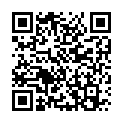# North Coast Synthesis Ltd.

## B♭+2+11+♯2Choose audio sample set:
MSK 007 Leapfrog VCF (patch; see in online store)
MSK 009 Coiler VCF (patch; see in online store)

Equivalent chord symbols: A♯+2+4+♯2, A♯+4+9+♯2, B♭+2+4+♯2, B♭+4+9+♯2, A♯+2+11+♯2.

Notes in this chord: B♭, C, C♯, D, F, E♭. Integer notation: {0, 1, 2, 3, 5, 10}.

Nearby chords (one less note): B♭+2+4, B♭+2+♯2, B♭+4+♯2, B♭m+2+4, B♭4+2+♯2, Cm4+2+♯1.

Nearby chords (one more note): B♭11+♯2, Cm11+♯1, B♭M11+♯2, A♭13-1+♯4, B♭+2+4+♯1+♯2, B♭+2+4+♯2+♯4.

Parallel chords (same structure, different root): C+2+11+♯2, D+2+11+♯2, E+2+11+♯2, F+2+11+♯2, G+2+11+♯2, A+2+11+♯2, B+2+11+♯2, C♭+2+11+♯2, D♭+2+11+♯2, E♭+2+11+♯2, F♭+2+11+♯2, G♭+2+11+♯2, A♭+2+11+♯2, C♯+2+11+♯2, D♯+2+11+♯2, E♯+2+11+♯2, F♯+2+11+♯2, G♯+2+11+♯2, A♯+2+11+♯2, B♯+2+11+♯2.

Experimental fretting charts for guitar standard EADGBE tuning (change tuning or instrument):